# Horizontal distance

The road has a gradient of 8%. How many meters will the road rise on a horizontal distance of 400m?

x =  32 m

### Step-by-step explanation:Did you find an error or inaccuracy? Feel free to write us. Thank you!Tips to related online calculators

#### You need to know the following knowledge to solve this word math problem:

We encourage you to watch this tutorial video on this math problem:

## Related math problems and questions:

• The railway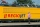The railway line has a gradient of 12 per mille. How many meters will it ascend to a horizontal distance of 4 km?
• ClimbFor horizontal distance 4.2 km road rise by 6.3 m. Calculate the road pitch in ‰ (permille, parts per thousand).
• Climb in percentageThe height difference between points A and B is 475 m. Calculate the percentage of route climbing if the horizontal distance places A, B is 7.4 km.
• Sales off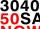Calculate what was the original price of the good, if the price after discount 25% and the subsequent rise of 20% is 400 USD.
• Cinema ticketsCinema sold 180 tickets this Thursday, which is 20%. Monday 14%, Tuesday 6%, Wednesday 9%, Friday 24%, Saturday 12%, and Sunday 15%. How many tickets were sold per week?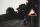A 5 km long road begins at an altitude 500 meters above sea level and ends at an altitude of 521 ASL. How many permille road rises?
• Brunette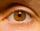Of the girls who attend primary school are 30% blondes and 70% brunette. 81% blondes and 19% brunette has blue eyes. How many girls attending school altogether if 470 girls has blue eyes?
• ThomasThomas lives 400 meters away from Samko, Robo from Thomas also 400 m and Samko from Robo 500. Anton lives 300 meters away from Robo further as Samko. How far away lives Anton from Rob?On a straight stretch of road is marked 12 percent drop. What angle makes the direction of the road with the horizontal plane?
• Percentage increaseIncrease number 400 by 3.5%
• Railway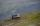Between points A, B, whose horizontal distance is 1.5 km railway line has 8promile climb. Between points B, C with horizontal distance of 900 m is climb 14promile. Calculate differences of altitudes between points A and C.
• Square - increased perimeterHow many times is the increased perimeter of the square, where its sides increase by 150%? If the perimeter of the square will increase twice, how much% increases the content area of the square?
• RailwaysRailways climb 7.4 ‰. Calculate the height difference between two points on the railway distant 3539 meters.
• Percents from percentHow much is 13% of 20% of 500 greater than 8% of 14% of 200?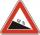The road sign informs the gradient is 10.9%. Calculate the angle at which the average decreases.Persons surveyed:100 with the result: Volleyball=15% Baseball=9% Sepak Takraw=8% Pingpong=8% Basketball=60% Find the average of how many like Basketball and Volleyball. Would you please show your solution?How many grams of salt should we dissolve in 400 g of water to get a 20% solution?